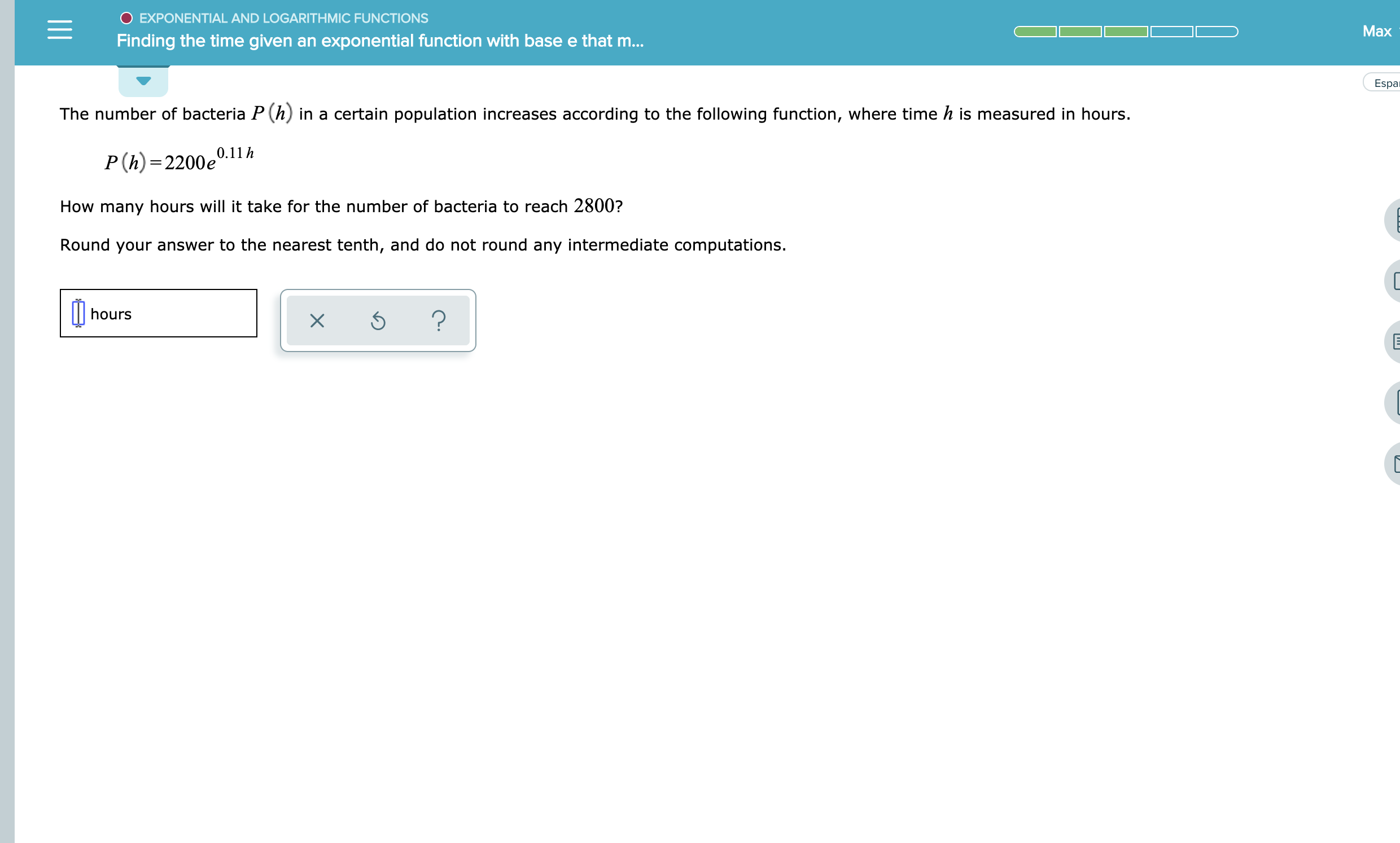EXPONENTIAL AND LOGARITHMIC FUNCTIONSМaxFinding the time given ant exponential function with base e that m...EspaThe number of bacteria P (h) in a certain population increases according to the following function, where time h is measured in hours.P (h)=2200e0.11hHow many hours will it take for the number of bacteria to reach 2800?Round your answer to the nearest tenth, and do not round any intermediate computations.hours?

Question

See attachmenthelp_outlineImage TranscriptioncloseEXPONENTIAL AND LOGARITHMIC FUNCTIONS Мax Finding the time given ant exponential function with base e that m... Espa The number of bacteria P (h) in a certain population increases according to the following function, where time h is measured in hours. P (h)=2200e0.11h How many hours will it take for the number of bacteria to reach 2800? Round your answer to the nearest tenth, and do not round any intermediate computations. hours ? fullscreen
Step 1

P(h) = 2,800 = 2,200e0.11h

Hence, e0.11h = 2,800 / 2,200 = 1.2727

Step 2

Take log to the base e on both side (represented by ln)

Hence, ln(e0.11h) = 0.11h = ln (1...

Want to see the full answer?

See Solution

Want to see this answer and more?

Our solutions are written by experts, many with advanced degrees, and available 24/7

See Solution
Tagged in

Calculus